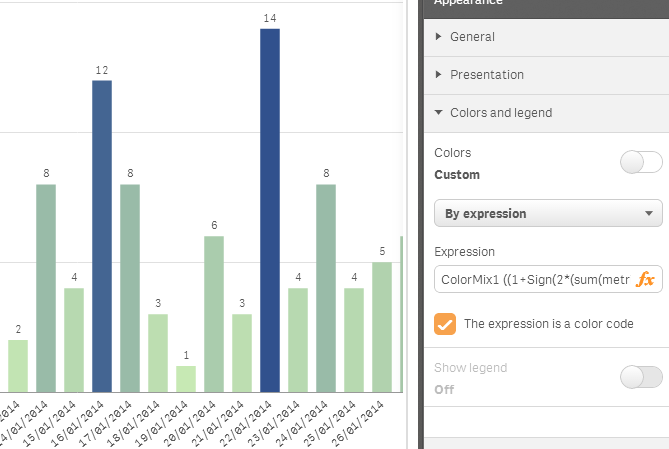# New to Qlik Sense

If you’re new to Qlik Sense, start with this Discussion Board and get up-to-speed quickly.

Announcements
Qlik Cloud Maintenance is scheduled between March 27-30. Visit Qlik Cloud Status page for more details.
cancel
Showing results for
Did you mean:Not applicable

Hi,

Can anybody tell me how can i apply gradient color to a bar chart in Qliksense. I am trying to modify the bar chart color in the sample Executive (Sales Analysis tab) dashboard provided but unable to get gradient effect with custom color. I would really appreciate any help on this.

Thanks.

1 Solution

Accepted SolutionsNot applicable
Author

Hi,

Yes you can use Colormix in a bar chart (and other charts) in Qlik Sense by using the Color by expression property. But, you better take advantage of the wizard for Colormix in QlikView and then paste the expression in Sense.5 RepliesCreator II

Hi,

there are default gradient colors in qliksense you can use that no need to go for custom colors.

If you want to use gradients other than default you can use color mix function in color expression. You can use qlikview to generate color mix expression. That will work in qliksense too

regards,

Prabhu AppuAnonymous
Not applicable
Author

Hi,

Are you trying to get a gradient within the bar, so each bar goes from light to dark, or across the bars, so the largest bar is dark and the smallest is light?

In terms of visualisation best practice, setting a gradient within the bar is not a great idea as it might convey a confusing message, and it is also not possible in Qlik Sense. Using colour gradients across bars using the ColorMix wizard is a good technique however.

Depending on the situation there might be other techniques you can look at. What are you trying to achieve or show with the gradient?Not applicable
Author

Thanks for the reply. I am trying to get gradient across the bars. I am doing a few user studies using colors hence i was trying to achieve Gradient for different colors. I am using this expression to generate a gradient but is not much helpful argb((Sum([Sales Quantity]*[Sales Price])*(255/(aggr(max(sum([Sales Quantity]*[Sales Price]),[Product Group]))))),255,0,0).

Let me see how i can use colormix....Not applicable
Author

Hi,

Yes you can use Colormix in a bar chart (and other charts) in Qlik Sense by using the Color by expression property. But, you better take advantage of the wizard for Colormix in QlikView and then paste the expression in Sense.Creator II

Hi Borja!

I know that your answer was sent 3 years ago. But i'm trying to solve my situation.

I used the Qlik View to color a a Pivot Table in Qlik sense, my expression is

ColorMix1 ((1+Sign(2*((SUM(RISCO_C_11_14)+SUM(RISCO_D_15_30))

/

before(sum({1}RISCO_A_EM_DIA)) -RangeMin (top(total (SUM(RISCO_C_11_14)+SUM(RISCO_D_15_30))

/

before(sum({1}RISCO_A_EM_DIA)) ,1,NoOfRows(total))))/(RangeMax (top(total (SUM(RISCO_C_11_14)+SUM(RISCO_D_15_30))

/

before(sum({1}RISCO_A_EM_DIA)) ,1,NoOfRows(total)))-RangeMin (top(total (SUM(RISCO_C_11_14)+SUM(RISCO_D_15_30))

/

before(sum({1}RISCO_A_EM_DIA)) ,1,NoOfRows(total))))-1)*Sqrt(Fabs((2*((SUM(RISCO_C_11_14)+SUM(RISCO_D_15_30))

/

before(sum({1}RISCO_A_EM_DIA)) -RangeMin (top(total (SUM(RISCO_C_11_14)+SUM(RISCO_D_15_30))

/

before(sum({1}RISCO_A_EM_DIA)) ,1,NoOfRows(total))))/(RangeMax (top(total (SUM(RISCO_C_11_14)+SUM(RISCO_D_15_30))

/

before(sum({1}RISCO_A_EM_DIA)) ,1,NoOfRows(total)))-RangeMin (top(total (SUM(RISCO_C_11_14)+SUM(RISCO_D_15_30))

/

before(sum({1}RISCO_A_EM_DIA)) ,1,NoOfRows(total))))-1))))/2, RGB(255, 34, 0), RGB(0, 255, 17))

The editor says it is correct, but not works.

Can you help me?

TksTags
Community Browser